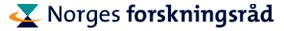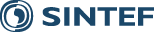Lectureurs and Topics

### Spectral element methods for incompressible flow

The emphasis will be on developing discretization and solution algorithms for solving the unsteady, incompressible Navier-Stokes equations. The applications will be selected from the laminar to the transitional flow regime.

• Governing equations
• Spatial discretization (the spectral element method)
• Poisson
• Stokes
• Convection-diffusion
• Navier-Stokes
• Temporal discretization
• Multi-step methods
• Operator splitting methods
• Segregated algorithms
• Solution methods
• Iterative methods
• Preconditioning
• Domain decomposition methods
• Fast diagonalization methods
• High Reynolds number flows (Re ~ O(1000))
• Transitional flows
• Filtering
• Grid generation
• Parallel processing
• Applications
• Blood flow
• Convection heat transfer
• (Free surface flow)
The above list is tentative, and the actual lecture topics and lecture order will be chosen and mixed at a later stage.

### Runge-Kutta discontinuous Galerkin methods for convection-dominated problems

• An overview of the development of Discontinuous Galerkin methods
• The Runge-Kutta Discontinuous Galerkin method for nonlinear hyperbolic problems.
• The Local Discontinuous Galerkin method for convection-diffusion problems.
• A unified analysis of Discontinuous Galerkin methods for elliptic problems.
• Discontinuous Galerkin methods for incompressible flows.

Published October 20, 2010